### 5263 - Vector Model Information Retrieval

Theory of Information Retrieval, Florida State University LIS-5263 (Fall, 2003)
Written by Rich Ackerman, September 25. 2003
http://www.hray.com/

## Introduction

This document contains explanations of some elementary mathematical concepts and shows their application in the development of the vector model of information retrieval as described in "Modern Information Retrieval" by Baeza-Yates and Ribeiro-Neto (1999). It was written in partial fulfillment of the requirements of the fall, 2003 class, "LIS-5263 - Theory of Information Retrieval," at Florida State University.

The discussion is divided into two parts; one covers basic math, and the second covers the math of the vector model. This document is meant to be used in conjunction with the textbook and other class material.

The first section covers essential mathematical building blocks:

• Logarithms
• Cosine
• Summation
• Dot Product (vector multiplication)

The second section explains the math behind the calculations required for the vector model:

• Inverse Document Frequency (idf)
• Normalized Frequency (f i, j )
• Weight Calculations

## Mathematical Building Blocks

### Logarithms: log(N)

The logarithm is the first mathematical function we need to understand. The vector model has equations like log ( N / n i). If you don't know what a logarithm is, then you cannot understand what that means.

First of all, what is a function? In its simplest form, a function is a computation that takes a number as input, performs a calculation on that input, and returns the value of the calculation. Consider the square function, "sq". sq(2) returns 4. sq(9) returns 81. So sq(N) just means to return the value of N * N.

Following that line of thinking, log (2) means to return the logarithm of the number 2. Log (N) means to return the value of the logarithm of the variable N, for whatever value N might have.

We know how to square a number but what is the logarithm function? How is it computed?

We won't go into the derivation but if you play with Google a little, you can see the types of values that the logarithm function returns. Google has a built-in calculator that we will use. Type "log 0.5" (without the quotes) into Google and you get -0.301029996. Try "log 95" and you get 1.97772361. Do a few more. What is log 6666 ? Just poking at random numbers gives us a little feel for the function, but an organized set of numbers reveals the true pattern:

 N log ( N ) 1 0 10 1 100 2 1000 3 10000 4

10 to the power of log (N) = N. So, if you have a number, and you take its logarithm, and then raise 10 to that power, you get back to your original number.

The chart shows that the logarithm function is useful in compressing numbers from very big to managable sizes; it's an "order of magnitude" reduction calculation. Also, we note that the log(1) is equal to zero. Both of these are useful and necessary in calculating weights, as we will soon see.

One more interesting characteristic of the logarithm function is that logs of values between 0 and 1 are negative numbers. For instance, log (0.5) = -0.301029996. A lot of times you'll see -log (X) in an equation. If X is a variable that ranges from zero to one, now you know why the negative sign may be there: the problem may demand that the number be positive. Putting in the negative sign will turn a negative value positive.

So now if you see log (N) in an equation, you have a handle on what it means and can estimate some values for it! A logarithm just measures the order of magnitude of a number N. It's just a smaller number substituting for the original one. And in the special case where N is one, then the log (N) will be zero.

### Cosine

The cosine is a trigonometric function with some properties that are very useful in analyzing vectors.

We are representing documents in our collection as vectors. We also represent the query as a vector. If we could somehow compare the query vector to the vectors of each of the documents, then we would be able to say which of the documents is "closest" to the query. This would gives us a ranking for our search. See the class notes for a good description of how that works.

So how do you rank the closeness of vectors? One way to consider (but it will get more complicated, of course!) is to just take the angle between them. If the angle is very small, then the two vectors are pretty close. If the angle is big, then they are not. Easy. However, if we want to do math on this and we are dealing with angles, we get a lot of weird numbers like 72 degrees. Just like the log function smooths out numbers, angles are easier to deal with if they are normalized to vary in a regular way... like between one and zero, for instance. 0 could mean "totally different" and 1 could mean "identical" with a full range of values (similarities) between 0 and 1.

Now, if we make a little chart of some angles and their cosines, we can see how the cosine smooths out the angles:

 Angle A Cosine (A) 0 1 30 .86 45 .70 60 .5 90 0

The table shows that when the angle between two vectors is very small, the cosine approaches 1. Indeed, if the two vectors match perfectly, the cosine equals 1. In our IR application, that would be a perfect match between the query vector and a document vector.. As the angle increases, the cosine (the degree of similarity between the vectors) diminishes until it reaches zero when the vectors are at 90 degrees.

For those who like pictures: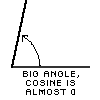In a coordinate system where every coordinate had equal weight, that might be an approximage measure of similarity. However, for our vector model, we want to value different coordinates (search terms) differently, so we have to make it a little more complicated. We'll do that pretty soon!

For now, we see that cosine is a function that measures a relationship between two vectors. The cosine of the angle between them approaches one as the vectors converge and approaches zero as the angle between the vectors approaches ninety degrees.

###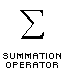Now we need to review the summation operator, pronounced "sigma." It looks scary but it isn't that bad!

The sigma is an operator, just like a multiplication sign or a division sign. It says to add together a series of numbers. When you see a multiplication sign, you know you are going to be multiplying numbers. When you see a sigma, you know you will be adding together a series of numbers.

The full expression looks like this:This symbol denotes the sum of the terms: a1 + a2 + a3+ ... + an. "a1" means the first number in the series. " a2 " is the second, etc. "an" is the final term in the series. You know lots of series: 1, 2, 3, 4, ... is a series. 2, 4, 6, 8, 10, ... is a series. 1, 3, 2, 4, 3, 5, 4, 6, ... is a series. "a1 + a2 + a3+ ... + an" and the sigma expression above are two different ways of saying the same thing.

There are a few variables associated with that expression that you need to understand as well. The variables determine how many terms get added and which members of the series are included:

• The variable k is the index of summation. The values of k run from 1 to n in the expression above.
• The a's are the terms that get added together. They can be very complex expressions, but they are always members of a series and they always get added together.
• The number 1 is the lower limit of summation. That tells you which term to start with.
• The variable n tells you when to stop, the upper limit of summation.

When you see an expression like that, the English translation would be "Take the summation from k equals 1 to k equals n of a sub k." (Finney et. al. 1995)

Let's look at some examples:

Example 1: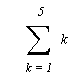In English: Take the sum of all k's from k=1 to k=5

Numerically: 1 + 2 + 3 + 4 + 5 = 15 ... fifteen is the answer.

Example 2:In English: Take the sum of the squares of k as k goes from k=3 to k=6

Numerically: 9 +16 + 25 + 36 = 86 ... 86 is the answer.

Example 3: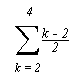In English: Take the sum from k=2 to k=4 of (k - 2) divided by 2

Numerically: 0 + 1/2 + 1 = 1.5 ... 1.5 is the answer.

So, a sigma just means "take the sum of this series" and when you see a sigma, you just figure out what the terms are, and then add them together. You'll always be adding them together; that's what it means.

### Dot Product

Fasten your seat belt! This section gets a little challenging, but it is the essence of how the vector model works. This section describes yet another mathematical operator.

When mathematicians (or physicists, or engineers, or librarians) want to multiply two vectors together, one way they do it is with an operator called a "dot product." Its symbol is a big huge fat dot: If you had two vectors A and B and wanted to multiply them together, you'd write A B. You'd pronounce that expression "A dot product B." The result of a dot product operation is a scalar (a single number), not a vector.

If the vectors are perfectly aligned, you calculate a dot product by multiplying their corresponding terms and adding the products together. There will be as many products as there are dimensions in the coordinate system. Thus, for a three dimensional vector, the dot product would be the sum (see, it's a scalar!) of the corresponding coordinates multiplied with one another.

If A = (a1 , a2, a3 ) and B = (b1 , b2, b3 ), then

A B = a1b1 + a2b2 + a3b3 (where a1b1 means the first coordinate in A times the first coordinate in B, etc.)

Example: If A was a vector (3, 4, 7) and B was a vector (9, 2, 1) then

A B = (3 * 9) + (4 * 2) + (7 * 1) = 27 + 8 + 7 = 42.

Hint: in our complex document world, there are N documents and so there are N terms in the vectors!

You may recognize this as a sum of a series and recall the summation operator:This just says that for two vectors, A and B, that are perfectly aligned, A B equals the sum of the products of the corresponding coordinates of the two vectors.

However, if vectors are not perfectly aligned, we need a fudge factor to account for the skew in their alignment. Thus the generalized formula for a dot product brings in our friend the cosine:

A B = |A| |B| cos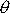wheremeasures the angle between the vectors A and B and |A| means the absolute value of the vector. [The derivation of this comes from the Law of Cosines but you can just believe that it is true!]

If you think about some cases, you'll see that this makes sense. When the vectors are pointing at right angles, cosis zero, so the dot product is zero. When the vectors are very close, the cosine approaches one and dampers it just a little. This is used in physics when calculating work: the vectors are force and distance, and the angle is the angle at which the force is applied. If you push a dresser with your shoulder so the force is parallel to the floor, it will slide easily (but will scratch the floor!) If you lift the dresser up and move it, it's a lot more work (but you don't scratch the floor!)

One variation of this will show up later. If we divide both sides of this equation by |A| |B|, we get:Since we reasoned earlier that cosine was a good proxy for measuring the similarity between vectors, now we have discovered a relationship that we can use to describe the similarity between two vectors. Using "sim (A, B) to mean that similarity, substitute that in for the cosine above and you get: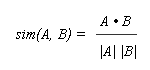This is the key mathematical expression we need for the vector model, because it provides a formula for calculating similarity between vectors in terms of those vectors. There are, however, a couple of other dot product formulas we need to see before we get into the vector model. This uses some algebra to make variations on the equations we've already seen.

Starting from A B = |A| |B| cos, if you substitute A for B (in other words, use the same vector for both A and B) we get:

A A = |A| |A| cosBut if it is the same vector, then it is lined up with itself, so the angle between them is zero, so the cosine of the angle equals 1.

Substituting 1 for cosgives us A A = |A| |A| = | A | 2

If we take the square root of each side we getRecalling that A B = a1b1 + a2b2 + a3b3 , we can see that A A = a1a1 + a2a2 + a3a3

But a1a1 is a12 so a more compact way of saying this is:

A A = a12 + a22 + a32

By substituting the series for the dot product, we get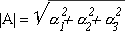Under the square root sign we see a series, the sum of the squares. Thus this equation can be written more compactly as:We will see very soon how this helps calculate the degree of match between a query vector and a document vector.

Well, I told you to buckle your seatbelt! This is all the background you need for dot products, and it is all the background you need to understand the vector model of information retrieval.

## Vector Model Calculations

The vector model of Information Retrieval relies on three sets of calculations. This model can work on selected index words or on full text. In the discussion below, it really doesn't matter.

The calculations needed for the vector model are:

1. The weight of each index word across the entire document set needs to be calculated. This answers the question of how important the word is in the entire collection.
2. The weight of every index word within a given document (in the context of that document only) needs to be calculated for all N documents. This answers the question of how important the word is within a single document.
3. For any query, the query vector is compared to every one of the document vectors. The results can be ranked. This answers the question of which document comes closest to the query, and ranks the others as to the closeness of the fit.

Throughout all the discussion, we need to have the basic vector model definitions at our disposal:

 N The total number of documents in the system. If your database contains 1,100 documents that are indexed, then N = 1,100. ki Some particular index term. ni The number of documents in which the term ki appears. In the case above (where N = 1,100), this can range from 0 to 1100. freq i , j The number of times a given keyword (ki ) appears in a certain document (document "j") w i , j The term weight for ki in document j. w i , q The term weight for ki in the query vector. q = (w 1 , q , w 2 , q , ... w t , q) The query vector. These are coordinates in t-dimensional space. There are t terms in the index system; the query vector has a weight for every one of them. (The book puts little vector signs over the variable name; I am bolding it instead). dj = (w 1 , j , w 2 , j , ... w t , j) A document vector. There are N documents in the system so there are N document vectors. Each one has a weight for each keyword in the indexing system. If the system is large, many of these values will be zero, since many keywords will not appear in the document vector for any given document. In mathematical terms, this is called "sparse". f i , j Normalized frequency of ki in dj dj A representative document, the "j th" one.

The vector model definition on p. 27 describes these terms (Baeza-Yates & Ribeiro-Neta, 1999). In addition, it explains the query vector and document vectors. These consist of coordinate sets where the coordinates are the weights for each term in the entire system. With very little discussion, the book then lays out this rather intimidating pair of equations to describe similarity between the vectors: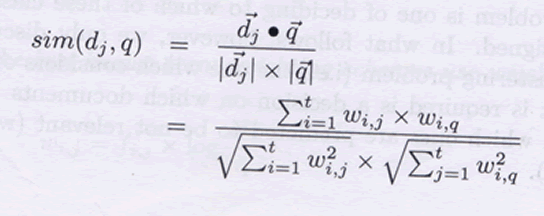We are going to work our way through this, and you will soon see what this means.

### Similarity

Starting back with our discussion of dot products, we remember that we could use cosine as a proxy for similarity between vectors, and arrived at this equation:Translated into English, that says that the similarity between two vectors is equal to the dot product of the vectors divided by the product of the absolute values of the vectors. In the vector model problem, we know that our two vectors are the query vector and a document vector. So, letting A be a document vector (dj ) and B be the query vector (q), we can substitute for A and B to get the starting point that the book uses:Now we use the dot product math that we learned earlier to expand this out. Why do we want to do that?

The basic problem is that the formula does not incorporate the weights of the terms in the query vector and the document vectors. We want to use the weights to find out which document(s) are closest to the query vector. To solve for the similarity, then, we have to use a series of equations to express this same formula in terms of the vector coordinates (the weights).

First, recall that:

A B = a1b1 + a2b2 + a3b3 from the definition of dot product above. This just means that to calculate the value of a dot product, you multiply the first coordinates together, then add them to the product of the second coordinates, then add that sum to the product of the third coordinates, and you keep going until you hit the end of the coordinates. You end up with a single number, not a vector.

Now, in our vector model, we are saying that A is the document vector, dj = (w 1 , j , w 2 , j , ... , w t , j)

and B is our query vector q = (w 1 , q , w 2 , q , ... , w t , q)

So we substitute for A and B, and expand out the terms, taking each term of dj , multiplying it by the corresponding term of q, and adding up all these products:

dj q = (w 1 , j * w 1 , q ) + (w 2 , j * w 2, q ) + ... + (w n , j * w n , q )

So we are starting to incorporate the weights of the terms here. Putting this back into our equation for similarity we get:Now what about that denominator, | d | | q |. What can we do with that? If we start with equation 2:and we substitute a document vector for A, we get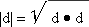for the document component

and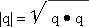for the query component

Substituting these into our monster equation, we have: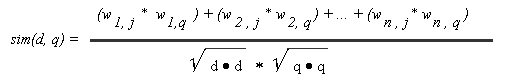Well, we already know how to get rid of dot products: expand them out into the sum of the products of corresponding terms. So we need to expand out the two square roots in the denominator.

Remember, though, that when you take the dot product of a vector with itself, you get a series of squares. If it is D D, the answer will be d1d1 + d2d2 + d3d3 which is the same as d12 + d22 + d32. For our document vector, that turns into a series of the sums of the squares of the weights:

dj dj = (w 1 , j * w 1 , j) + (w 2 , j * w 2, j) + ... + (w n , j * w n , j)

or

dj dj = w 1 , j 2 + w 2 , j 2 + ... + w n , j 2

From our little work on sigma, we recognize that as the sum of the squares, a very simple mathematical series. Since for the document vector the terms are the weights w i , j we end up with: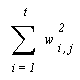The query vector similarly reduces to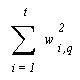Each of these looks intimidating, but the meaning is pretty simple. You just work through the coordinates one at a time. For each one, you square it and add it to the running total.

So, putting our two sigmas back into the equation gives us: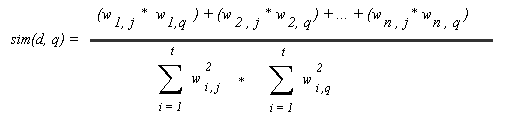Now are almost there. One last substitution and we'll be where the book ended up. The numerator is also a series that can be expressed through sigma notation:

(w 1 , j * w 1 , q) + (w 2 , j * w 2, q) + ... + (w n , j * w n , q)

Looking at this we see a series being summed, where each term is the product of the weight in the document vector times the weight in the query vector for the given keyword. In sigma notation that is: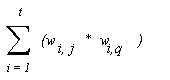and if we put that back into our equation we get the intimidating equation in the book: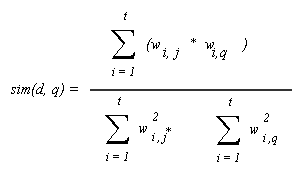Now, though, we know what it means and where it came from. We could even calculate some sample values if we felt so inclined. Given a document vector and a query vector, all we have to do is multiply some pairs of weights, add up those products, do a little division, and we have a measure of similarity. It looks hard but it is really pretty easy. The class notes give a numerical example, and my vector utility program shows the values and how these sums are calculated for sample queries you enter.

### Word values and weights

Up to this point we have thrown around a lot of expressions like (w n , j * w n , q) while ignoring exactly what those weights are. We have seen that using weights we can calculate the similarity between vectors, and thus the closeness of fit between a document vector and a query vector. By comparing the query vector to many document vectors, we can rank the documents as to the "goodness" of the fit against the query. But just what are those weights and where do they come from? The next sections describe how the weights are calculated. There are actually a lot of weights that need calculating!

One set of weights that we need, obviously, are the weights used in the query vector. The query vector contains every index term usable in the document set. The weight in the query vector reflects the keyword's importantance in the context of the entire document set. When N is large, this set of weights would probably get fairly stable. A new document could be added to the document set without significantly changing values in the query vector.

The second set of weights that we need is potentially enormous. For each of N documents in the document set, we need to calculate a weight for every index term in that document. Each document has a document vector containing the weight for every index term appearing in the document. If you have 1000 documents and 1000 index terms then you need to calculate 1,000,000 weights. Every time you add a new document to the document set, you need to calculate a set of weights for the words in the new document.

What is it that makes a word important to a search? It can be important in two contexts: in the setting of the original document, and in the context of the entire collection. A word that appears in every document, for instance, would have no value across the document set. To calculate keyword weights, then, we need a way to combine the importance of a word in a document and to measure the importance of a word in the entire document set. Once we have those values, we can calculate the weights. A table shows how these factors combine in general terms:

 Importance in document Importance in document set Weight High High Very high Low High Medium High Low Medium Low Low Very low

Information retrieval specialists use different methods for calculating document vector weights than for calculating query vector weights, and have a variety of techniques of measuring word importance in different contexts. In general, though, they use statistical techniques incorporating word frequency analysis to calculate these values. We will look at the frequency algorithms included in the textbook and then finally see how they are combined into weights.

### f i, j : Normalized term frequency (tf) of ki in d j

The first frequency we examine is the term frequency of a word within a document. We are going to learn how to calculate the normalized frequency of ki in d j.

Normalization is a mathematical process of smoothing or confining to a set range. As explained in the textbook, a raw count of frequency is essentially useless. In a very large document, a rare word may still appear 25 times. A small document in the same document set may have no words that occur 25 times. How can you compare those counts? We need to normalize the frequency count, so that it measures how rare a word is relative to its document regardless of the size of the document.

One way to do that is to compare the number of times a given word appears (freq i , j) to the number of times the most popular word in the text appears (max (freq l, j)). This is a way of scaling down the numbers and adjusting for the raw text size. If you divide them, you get a very controlled range of values, less than or equal to one. So we quickly get the formula in the book:This just says that the normalized frequency for a given word in a given document ( f i , j ) is equal to the raw frequency of the word in the document (freq i , j) divided by the raw frequency of the most common word in the document (max l freq l , j )

To see how nicely this normalizes the raw frequency counts, look at an example. As always, freq i , j is the raw count of a given term ki in a given document d j . Furthermore, we will pretend we have computed the value of freq i , j for all the terms in d j and know the most common word, max (freq i , j). Often that will be the word "the"; here we pretend it occurs 100 times.

 word freq i , j max (freq i , j) f i , j interception 1 100 .01 resolution 10 100 0.1 of 50 100 0.5 the 100 100 1

Again, "the" appears 100 times and is the most popular phrase. That earns it a normalized frequency of 1.0. The word "resolution" appeared ten times in this document, so its normalized frequency is 10/100 or 0.1. The word "interception" occurs but once and has a normalized frequency of .01.

In general, we see that common words would have higher normalized frequencies and rarer words would have lower normalized frequencies. Zipf's law predicts that there will be few words close to one and a lot of words in the very low range with a fair number in the middle, but that is a different topic. Finally, for a word in the larger document set but not contained in the document under examination,
f i , j would be equal to zero, since freq i , j = 0 and that is in the numerator of the normalized frequency.

So that's what normalized frequency is: a measurement of frequency ranging from zero to 1 for each term in a document. This formula assigns a higher value to words that appear more often than to words that appear less often; in some sense, the more common words are more important or valuable than the words that only show up once or twice.

### Inverse document frequency

Now we need to come up with a measure of frequency in the context of the entire document set. This is called the "inverse document frequency" and is given by the formula idf = log (N/ni). What does it mean and what's that formula all about?

First, let's consider that "inverse" part. Unlike the case of normalized frequency within a document, in the context of the entire document set we want we want the importance of a word to go down as the frequency of the word goes up. When the word appears in every document, we want this value to be zero. After all, if it appears in every document, it is worthless as a differentiator between documents. When the word only appears in a few documents, we want this to have a high value. Hence, the inverse: high count, low value; low count, high value. This is just the opposite of the normalized frequency.

So how do we put a number on this? Remember the definitions:

N is total # of documents
ni is # of documents in which the search term of interest (ki) appears

We can make a ratio of N divided by ni, (N / ni,) that will express this inverse relationship pretty nicely.

To see it, make a little table:

 N ni N/ni Discussion 1000 1 1000 Low count, high value! If the word only shows up once, the ratio turns out to be a very big number. Hmm, if we used this for a weight it might dominate too much. 1000 100 10 This is a reasonable number, perhaps... 1000 500 2 High count, low value. 1000 1000 1 Oops! We said that when the word appears in every document, we wanted the weight to be zero. Here our inverse ratio is equal to one. This is a problem.

So,we are close, but we have some problems. How do we fix the problems with our inverse ratio? We will use the logarithm function to normalized the values.

 N ni log(N/ni) Discussion 1000 1 3 Well, 3 seems like a nice number to use as a weighting factor for a really rare word. 1000 100 2 I like 2 too 1000 500 .301029996 If a word shows up in half the documents, it isn't worth much... hmmm, .3 sounds pretty good. 1000 1000 0 Bingo! We said that when the word appears in every document, we wanted the weight to be zero. Look at this! It's gonna work!.

So this looks like a pretty good idf. It is zero when the word appears in all the documents, and scales up slowly even as our collection size gets enormous.

To use a diving analogy, the "idf" is comparable to the "degree of difficulty" of a dive. The idf of a keyword is constant across a document collection. It is only needs to be calculated once for a given set of documents. The degree of difficulty of a dive does not change as different divers do it, and the idf of a search term does not change as the keyword is used in searches on different documents.

### Weights

Now, finally, we get to calculate weights!

We got a preview earlier of how we want weights to behave. If we add some sample data we see how well a simple multiplication of factors will work in creating weights:

 Normalized frequency Inverse document frequency Weight Sample nf Sample idf Sample weight (nf * idf) High High Very high .9 1.2 1.08 Low High Medium .2 .9 .18 High Low Medium .85 .04 0.034 Low Low Very low .15 .09 0.0135

As illustrated, since both terms have been normalized, we can simply multiply them together to get one weight value that follows the overall rules in the table.

This, in fact, is the formula recommeded for calculating document weights:This just says that the weight for ki in dj equals its normalized frequency (nf) times its inverse document frequency (idf). It will work well for both document weights.

For use in a query weight, of course, the normalized frequency would need to be calculated over the entire document set, not just a single document. The text suggests an enhancement in the calculation of the query vector: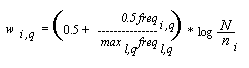This is saying the weight of term i in query vector q equals the normalized frequency of the search term in the "text of the information request q". Since this would usually be a low number, there is a fudge factor of 0.5: the recalculated normalized frequency gets half weight and a free "0.5" value is added in. This would increase the value of a search phrase that was entered more than one time. If you really wanted to find "jump" you could search for "jump jump jump" and this would increase the value of that phrase. I have my doubts about the efficacy of that as a user interface.

In my test software, I used the unmodified normalized term frequency for the query vector weight. Looking at the suggested term above, it seems as if the left multiplicand would be nearly constant.

### Conclusion

The vector model of Information Retrieval is a powerful tool for constructing search machinery. This discussion provided an introduction to the mathematical concepts required for understanding the vector model and showed the application of those concepts in the development of the model.

### References

Baeza-Yates, R. & Ribeiro-Neta, B. (1999). Modern information retrieval. New York : ACM Press.

Finney, R., Thomas, G., Demana, F., & Waits, B. (1995). Calculus : Graphical, numerical, algebraic. New York : Addison-Wesley Publishing Company.